# How to create guess number games using Python

In this article, we will create two simple games using Python code. Readers can use any Editor or IDE to create games, such as Pycharm and Jupyter Notebook. Furthermore, I assume that readers already set up their Python environment. In this case, I will use Visual Studio to be my IDE.

The first game is to guess a number between 1 to 100. Each guessing number will become a minimum or maximum. The person who guesses the number will become a loser.

The second game is to guess a number between 1000 to 9999. This random number won’t have duplicated. Each guessing will show A and B. A means current number and location. B means current number but wrong location.

First game:

The first part is to import the random package into the IDE because we will use randint() function to generate a random number. The 1 and 100 in the randint() function equal the minimum and maximum.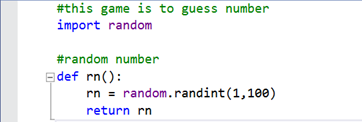First game — Part 1: random number definition

The second part is the main part. There are three points which should be careful.

The first point is that the input() function should be included by the int() function. According to the step, we can limit the input number in the integer type.

The second point is the while loop. This loop can keep running after the random number equals the input number.

The third point is the two variables. In order to shorten the range, we should set two variables to be minimum and maximum. Furthermore, use if-else statement to replace previous numbers when a player enters a number.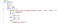First game — Part 2: main definition

The final part is to run the main definition. I use the print() function to show rules for players. Also, I only need to use the main() and rn() function to run all code because I use the def function to define my codes.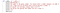First game — Part 3: main code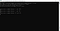First game — Part 4: Result

Second game:

The first part is the same as the first game to use the def function to generate a random number. However, we will change a little bit. In this case, we will generate a random number between 1000 to 9999. Furthermore, this random number can’t have repeated.

We use the sample() function to generate 4 digit numbers in 0 to 9 randomly. Secondly, We use the str() function and for loop to convert 4 digit numbers into a string. Finally, we convert the string data type to integer data type by int() function.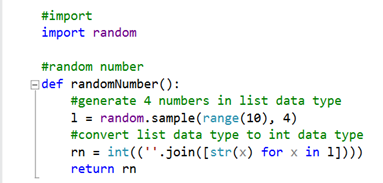Second game — part 1: Random number definition

The second part is the main part to divide numbers into digits and compare them one by one.

The first step is to use the division and modulus operators to divide the random number into 4 digits. Furthermore, we should build a list variable to save digits separately because it is easy to compare in for loop.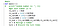Second game — part 2: Separate random number

The second step is to write the input() function into the while loop because players can guess numbers until success. Moreover, we should divide the guessing number into 4 digits because all guessing numbers are different in a game.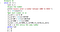Second game — part 2: Separate guessing number

The third step is to compare the random number and the guessing number. First for loop is to generate the parameter of A. We can catch this parameter easily when we use if statement. Second for loop is to calculate the parameter of B. According to the ch_B.append() function, we only catch the digit when they don’t fit the A condition. Thus, the calculation in the second for loop excludes the digit when it fitted A condition. We use double for loops here because we should compare digits one by one. For example, A -> A,B; B -> A,B.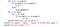Second game — part 2: Compared two numbers

The final part is the main code. As same as the previous game, the main code are simple because all codes are coded into the def function.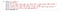Second game — part 3: Main code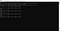Second game — part 4: Result

If you want to download those code files directly, please click this link below:

## More from Chen-Hua Wang (Danny)

A graduate student